[BACK]
 Computers, Materials & ContinuaDOI:10.32604/cmc.2021.013757Article

Analysis of Silver Nanoparticles in Engine Oil: Atangana–Baleanu Fractional Model

1Department of Mathematics, City University of Science and Information Technology, Peshawar, 25000, Pakistan
2Computational Analysis Research Group, Ton Duc Thang University, Ho Chi Minh City, 70000, Vietnam
3Faculty of Mathematics and Statistics, Ton Duc Thang University, Ho Chi Minh City, 70000, Vietnam
4Department of Mathematics, College of Science Al-Zulfi, Majmah University, Al-Majmah, 11952, Saudi Arabia
5Department of Mathematics, College of Arts and Science, Prince Sattam bin Abdulaziz University, Wadi Al-Dawaser, 11991, Saudi Arabia
Received: 20 August 2020; Accepted: 28 September 2020

Abstract: The present article aims to examine the heat and mass distribution in a free convection flow of electrically conducted, generalized Jeffrey nanofluid in a heated rotatory system. The flow analysis is considered in the presence of thermal radiation and the transverse magnetic field of strength B0. The medium is porous accepting generalized Darcy’s law. The motion of the fluid is due to the cosine oscillations of the plate. Nanofluid has been formed by the uniform dispersing of the Silver nanoparticles in regular engine oil. The problem has been modeled in the form of classical partial differential equations and then generalized by replacing time derivative with Atangana–Baleanu (AB) time-fractional derivative. Upon taking the Laplace transform technique (LTT) and using physical boundary conditions, exact expressions have been obtained for momentum, energy, and concentration distributions. The impact of a number of parameters on fluid flow is shown graphically. The numerical tables have been computed for variation in the rate of heat and mass transfer with respect to rooted parameters. Finally, the classical solution is recovered by taking the fractional parameter approaching unity. It is worth noting that by adding silver nanoparticles in regular engine oil, its heat transfer rate increased by 14.59%, which will improve the life and workability of the engine.

Keywords: Jeffrey fluid; silver nanoparticles; engine oil; Atangana–Baleanu fractional derivatives; Laplace transform technique

1  Introduction

Fluids that show non-linear relation between shear stress and shear strain are termed as non-Newtonian fluids e.g., castor oil, engine oil, colloidal solutions, and blood, etc. The non-Newtonian fluid has different rheological behavior than Newtonian fluids and has very substantial uses in the area of engineering, medical sciences, industries, electrochemistry, and mechanics . Due to the mentioned significance, it gathered great attention from researchers and scientists. Jeffrey fluid model has two generalized parameters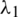and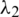. The models of Newtonian and second-grade fluid can also be recovered from it by neglecting the effects of their generalized parameters. Due to the above-stated facts, the Jeffrey fluid model has been dealt with by many researchers . After further examination, researchers reported that the thermal transport properties of the considered regular fluid are low. To boost the heat transport properties of regular fluids, researchers and scientists used the idea of suspended solid particles in the assumed conventional base fluid. As solid particles possess high heat transport properties as compared to regular fluids, it consequently enhances the heat transport properties of the base fluid. Keeping in view the above-addressed features of the solid particles, Maxwell , in 1873, used micro-sized solid particles in regular fluids. However, the significant blemish of microfluid was smaller scale estimated particles that typically stuck in the microchannel that block the apparatus. Besides, microparticles settled quickly in the base liquid and made issues of clogging, pipe disintegration, and surface wear. To circumvent the flaws of micro-sized solid particles in 1995, Choi et al. , introduced the idea of dispersing of nano-sized solid particles in conventional fluids to augment the thermal transport features of the conventional fluids. The resultant fluid that is obtained from the suspension of nanoparticles in the base fluid is referred to as nanofluid. Nanoparticles have widespread uses in different zones, for example, cooling power, vitality sparing, and medicate conveyance, and so forth. Different studies have been reported on the uses of nanoparticles . From the analysis, the researchers came to the point that nanoparticles are more stable and increase the conductive properties than micro size particles. Researchers also highlighted that nanoparticles decreases the problem of sedimentation and increase the stability of the regular fluid. Ali et al.  discussed the impact of silver nanoparticles in regular engine oil. Several other studies regarding nanoparticles applications can be seen in . By keeping in view the importance of nano-particles.

The study of magnetic properties of those fluids which allow electric current is known as magneto-hydrodynamic. Saltwater, engine oil, electrolytes, kerosene oil are examples of MHD fluid. The core work on MHD has given by Alfvén , and for this contribution, he got Nobel Prize in 1970. Magnetohydrodynamic or MHD for short, is the study of these motions in the case where the fluid can be treated as a continuum. On the basis of the above applications, Hayat et al.  developed a series form solution via the Homotopy analysis method for second-grade fluid. They considered the fluid in between two porous walls under the consideration of binary chemical reaction  studied the Maxwell fluid flow in a rotatory coordinate system. Seth et al.  also discussed unsteady electrically conducted fluid in a rotating channel and observed that fluid flow shows acceleration/deceleration when the magnetic field is varied. Abelman et al.  found a numerical solution for the time-independent flow of 3rd-grade fluid. Ali et al.  investigated the effect of the magnetic field on fractionalized Brinkman-type fluid flowing in a heated system. The authors modeled their mathematical model by inserting relative constitutive relations, and for their solution, they used the tool of Laplace transformation. For the interpretations of inserted parameters, they sketched the graphs. In their study, they highlighted that the magnetic parameter decline the velocity profile due to Lorentz forces occurs in the fluid. Khan et al.  inspected the effect of wall shear stress on conducting fluid flow for Newtonian fluid with variable ramped temperature.

Due to multidimensional features, the non-integer order calculus is fascinating to the attention of scientists and researchers . Fractional calculus is an important and fruitful tool for describing many systems, including memory effects. In the last few years, fractional calculus is used for many purposes in various fields, such as electrochemistry, transportation of water in ground level, electromagnetism, elasticity, diffusion, and in conduction of heat process . In 2015, Caputo et al.  worked together in the field of non-integer order calculus and presented a new expression for the fractional non-integer order derivative with the non-singular kernel. So keeping in sight the importance of the CF operator, many researchers used the CF operator in their studies such as  examined the MHD flow of Walter’s-B fluid by using the non-singular operator of CF. Several other studies regarding the uses of CF operators can be found in . But despite many properties, the kernel of the CF operator found local, and due to this deficiency, it was not remain reliable for describing the memory effect. So to fill the gap and solve this issue occurred in the CF operator, in the year 2016,  modified the operator of CF derivative by utilizing the Mittag–Leffler function and made its kernel non-singular and non-local. AB fractional operator has the beauty that it gives information about the original function, and one can also achieve the original function as well by letting the parameter of the fractional operator tends to zero. So due to the worth mentioned properties and advantages of AB fractional operator,  inspected the influence of thermo-diffusion on Jeffrey nanofluid in a rotating frame. They formed nanofluid by dispersing the metallic silver nanoparticles in regular Mobil oil. They have been acquired that velocity boosts up for increasing the magnitude of the fractional parameter. Jan et al.  discussed the impact of molybdenum disulfide nano-size particles in engine oil using the Atangana–Baleanu fractional model. Saqib et al.  acquired an exact expression for generalize Casson nanofluid, using AB fractional derivative.

Keep in mind the above literature review; we find a gap that no one has investigated Jeffrey nanofluid flow in a rotating frame with time-dependent temperature and concentration in initial and boundary conditions. To fill this gap, we considered fractionalized Jeffrey fluid flow in a rotating system. The governing equations containing the system of PDEs are developed by following the assumption of Boussinesq’s approximation . The classical model of the considered problem has been fractionalized via Atangana–Baleanu fractional operator. The mathematical tool of the Laplace transform technique has been utilized to acquire the solution mathematical model. The involvement of the constructive parameters on fluid motion, heat distribution, and concentration profile has been sketched. Change in the rate of heat and mass transfer was found numerically and computed in tabular form.

2  Mathematical Modeling and Solution

Consider a Cartesian coordinate system for unsteady electrically conducted free convection flow of an incompressible Jeffrey nanofluid past over an infinite rigid plate saturated in a porous medium under the effect of transverse magnetic field Bo. The plate is taken vertically upward in the direction of the x-axis. The rotation with constant angular velocity “” of the fluid is assumed about z-axis, which is normal to the plate, as shown in Fig. 1.Figure 1: The geometry of the flow

At time t = 0 both the plate and fluid are immobile. At t > 0 the plate is disturbed with cosine oscillation and transmits the disturbance to the fluid, which causes the fluid motion. Temperature and concentration of the fluid at time t = 0+ is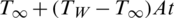andrespectively. Where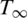and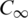represent surrounding temperature and concentration, respectively. TW and Cw represent wall temperature and wall concentration, respectively. Keeping in view, the above consideration applying Boussinesq approximation and using Tiwari and Das nanofluid model, the momentum, energy, and concentration equations are governed by:where F1 = u + vi is a complex velocity. Energy and concentration equations are: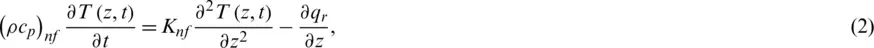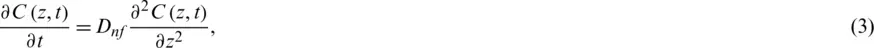pertinent initial and boundary conditions are: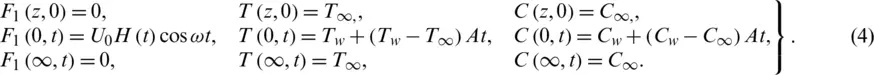Nanofluid expressions contained in the mathematical model are given in :

The radiative heat flux is given as: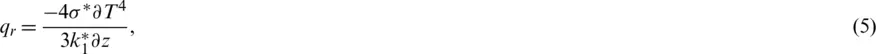Utilizing the Taylor expansion, the T4 can be written as: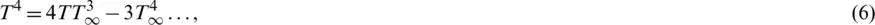substituting Eq. (6) into Eq. (5), and differentiate with respect to “” we get: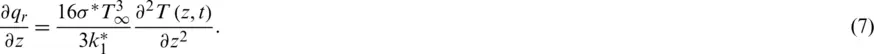The thermal transport properties of the base fluid and nano particles are given in Tab. 1.

Table 1: The heat transport properties of Engine oil and Silver nanoparticle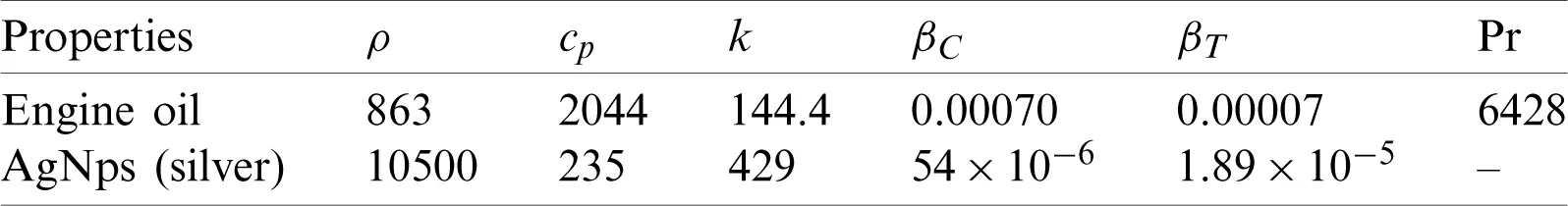The non-dimensional variables are: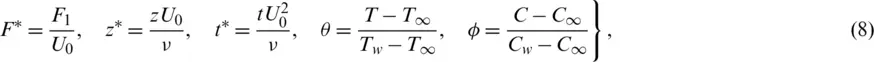Incorporating Eqs. (7) and (8), the governing equations will take the form;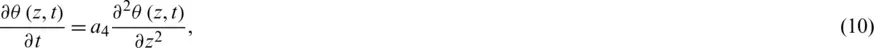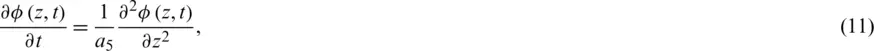andIn dimensinoalization process the constants we get: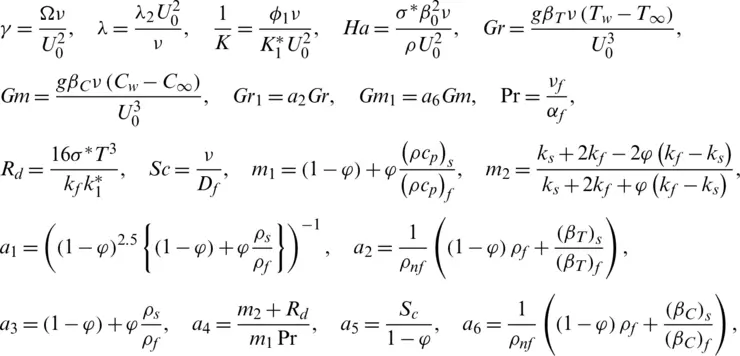The termandrepresent rotation parameter, Jeffrey fluid parameter, Permeability parameter, Hartman number, thermal Grashoff number, Mass Grashoff number, radiation, and Prandtl number, respectively.

2.1 Fractional Model

To fractionalized the ordinary model of the considered problem given in Eqs. (9)(11) to AB fractional model, we utilized the operator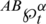instead of, and get: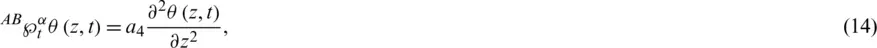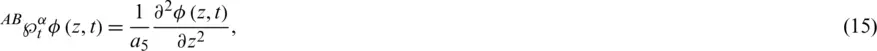where AB fractional derivative  is :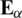is Mittag–Leffler function.

2.2 Solution of the Problem

Using the Laplace transformation on Eqs. (9)(11) and utilizing the relevant initial conditions from Eq. (12), we get: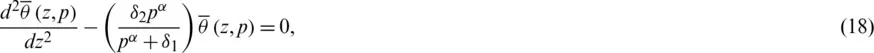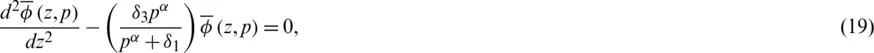with transformed boundary conditions as: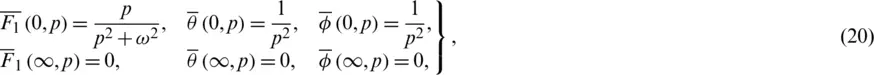whereusing conditions stated in Eq. (20) the solutions of Eqs. (17)(19) will be: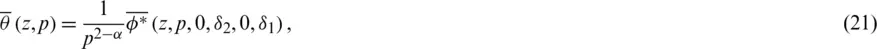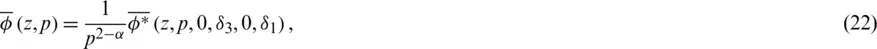and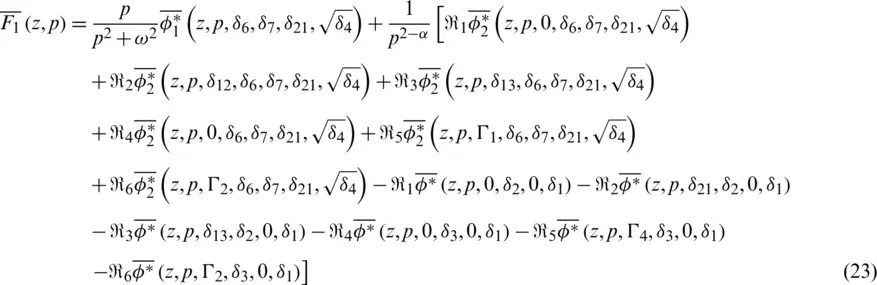where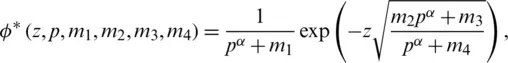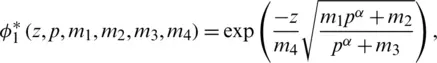andInverting the Laplace transform on Eqs. (21)(23), we get: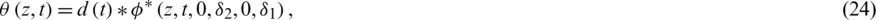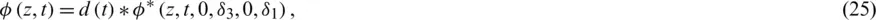andwhere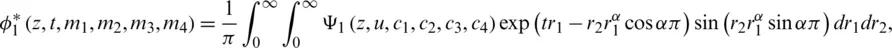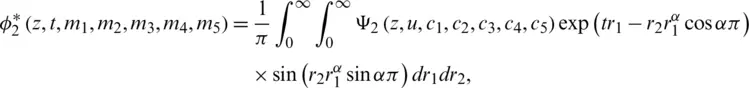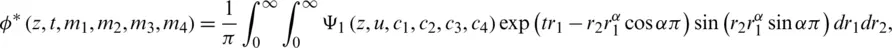in which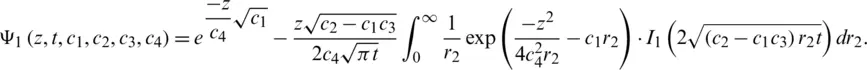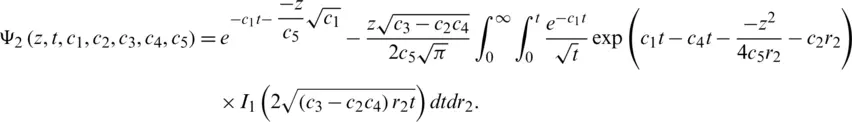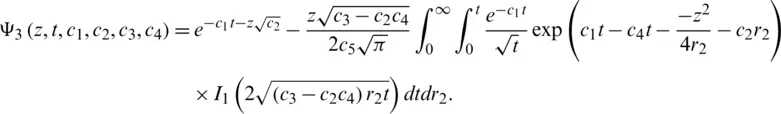And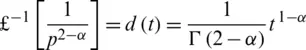2.3 Classical Exact Expression

Forand using the following property,the exact expression for velocity distribution obtained in Eq. (24) reduced to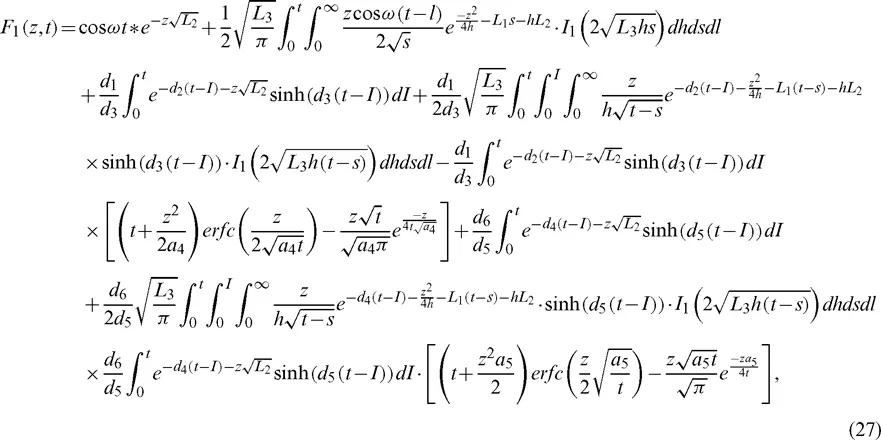where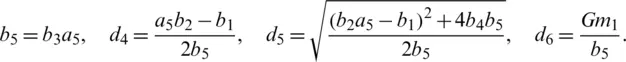3  Nusselt Number and Sherwood Number

In the non-dimensional form, the Nusselt number and Sherwood number are presented as: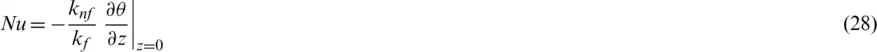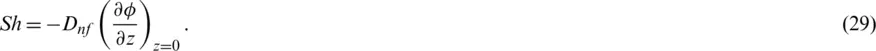4  Results and Discussion

This portion of the article devote to the physical arguments and detailed descriptions of the outcomes. Using relative constitutive equations, the governing system of PDEs is formulated. Nano-liquid has been made by the distribution of nano-silver in Mobil oil. The classical model of electrically conducted, incompressible Jeffrey nano-liquid is transformed into AB fractional model. Exact solutions have been gotten for the governing equations. To check the impact of inserted parameters on fluid motion, various sketches are portrayed.

The impact of Gr and Gm has been sketched in Figs. 2 and 3. Both the sketches show enchantment in the velocity profile for larger values. This happened due to a decrease in the density and increase in the bouncy forces which leads to speed up the velocity. The effect of Jeffrey fluid parameter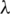and volume fractioncan be reported from Figs. 4 and 5. When the values of the material parameter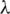and volume fraction of nanoparticles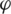rise, the viscous forces in the fluid increase; as a result, the friction force between the fluid particles increases, which leads to slow down the velocity of the fluid. It is concluded from Fig. 6 that for larger values of magnetic parameter Ha, the momentum of the fluid decelerates. A rise in the magnitude of Ha tends to increase the Lorentz forces which works as a drag force and retards the fluid motion. Moreover, the effect of angular rotationis drawn in Fig. 7. It can be noted from the sketch that velocity decreases for a large value of. From the mathematical relation ofit can be seen thatit has a direct variation with viscous forces of the fluid so larger the magnitude of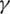the fluid motion decrease.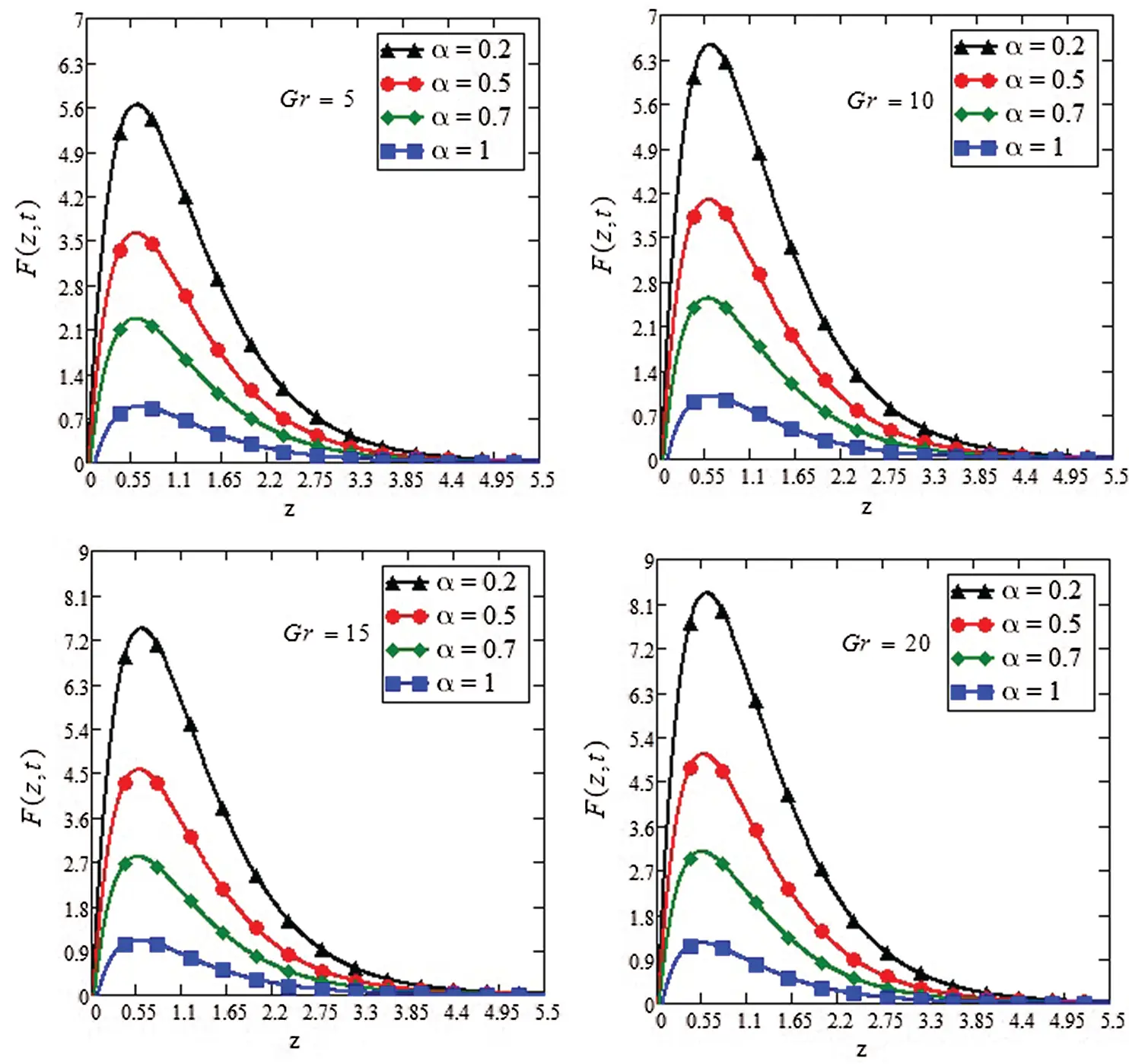Figure 2: Effect of thermal Grashof number Gr on the velocity profile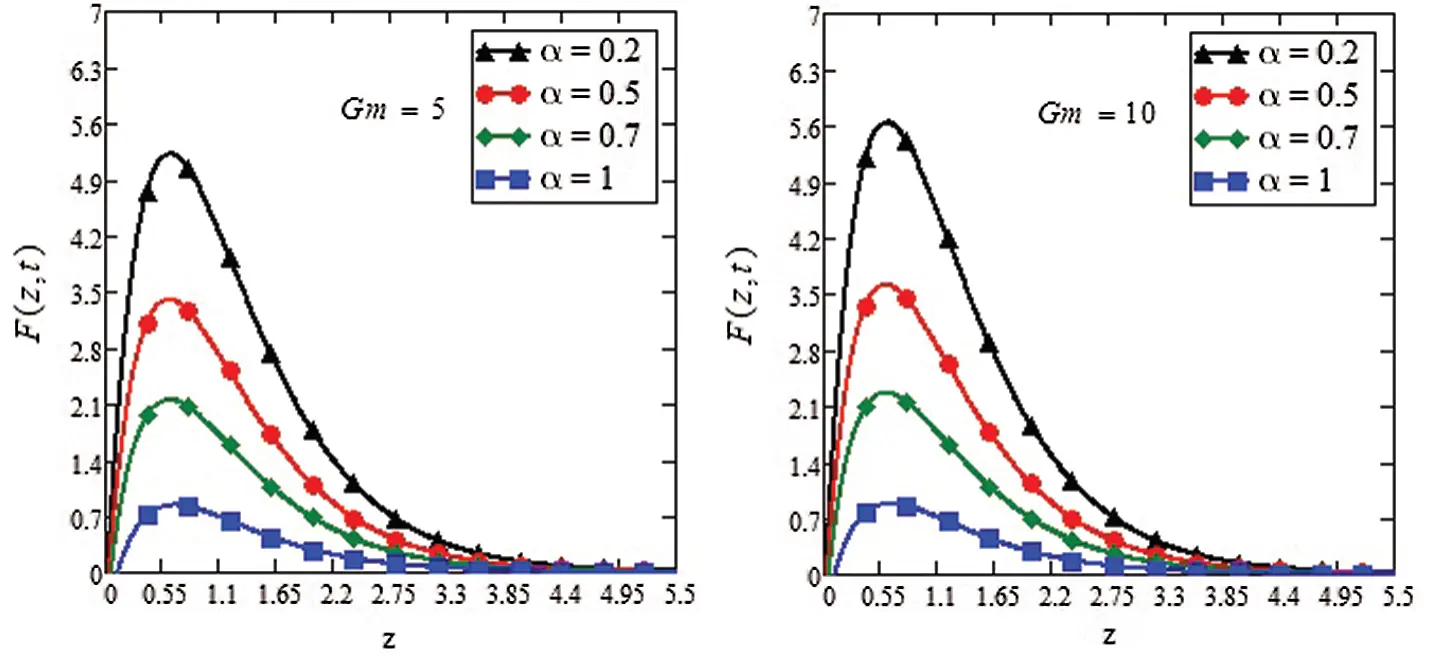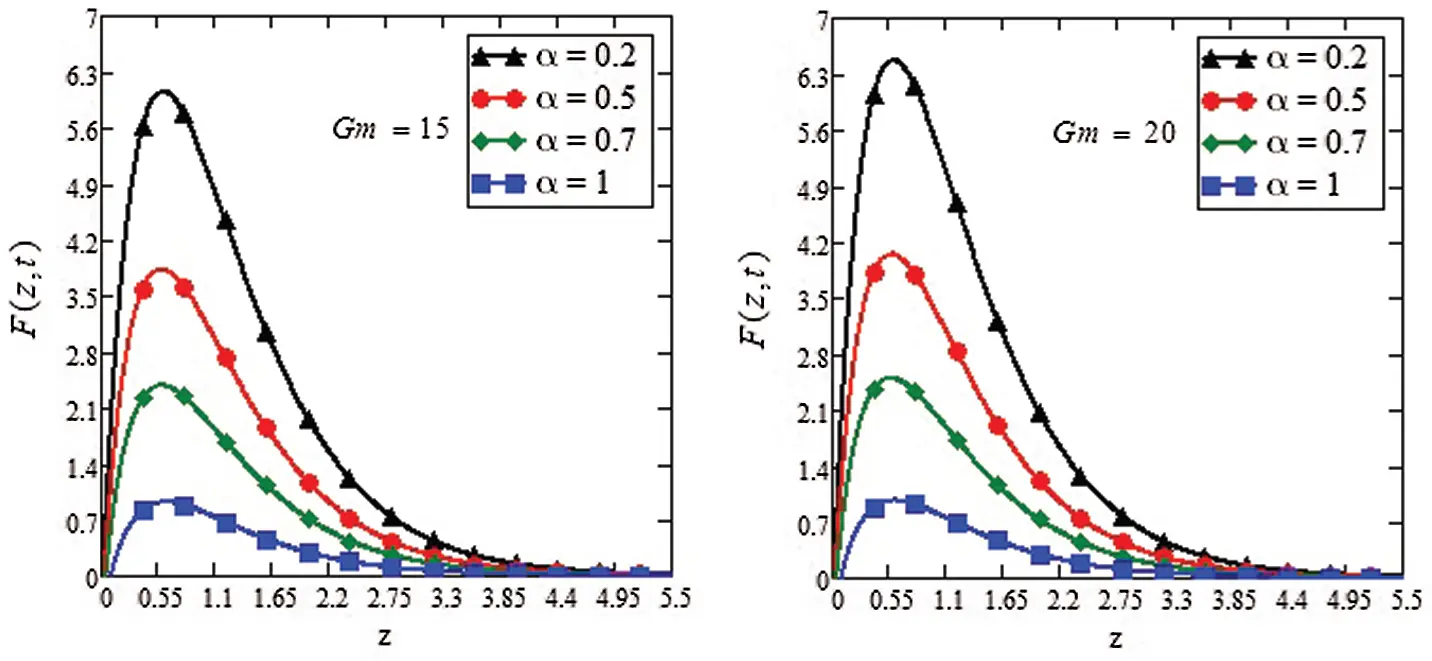Figure 3: Effect of mass Grashoff number Gm on the velocity profile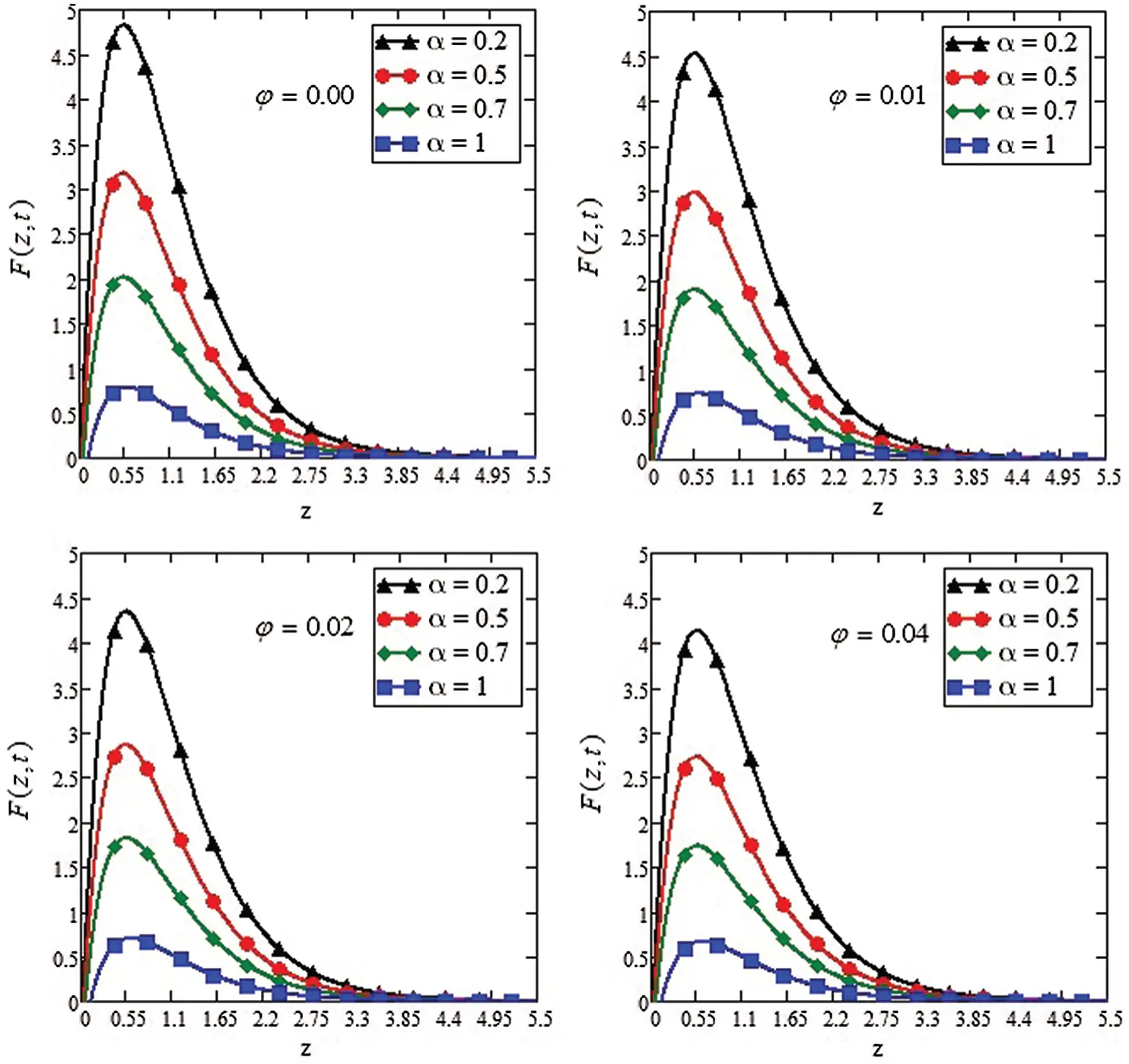Figure 4: Effect of retardation time parameteron the velocity profile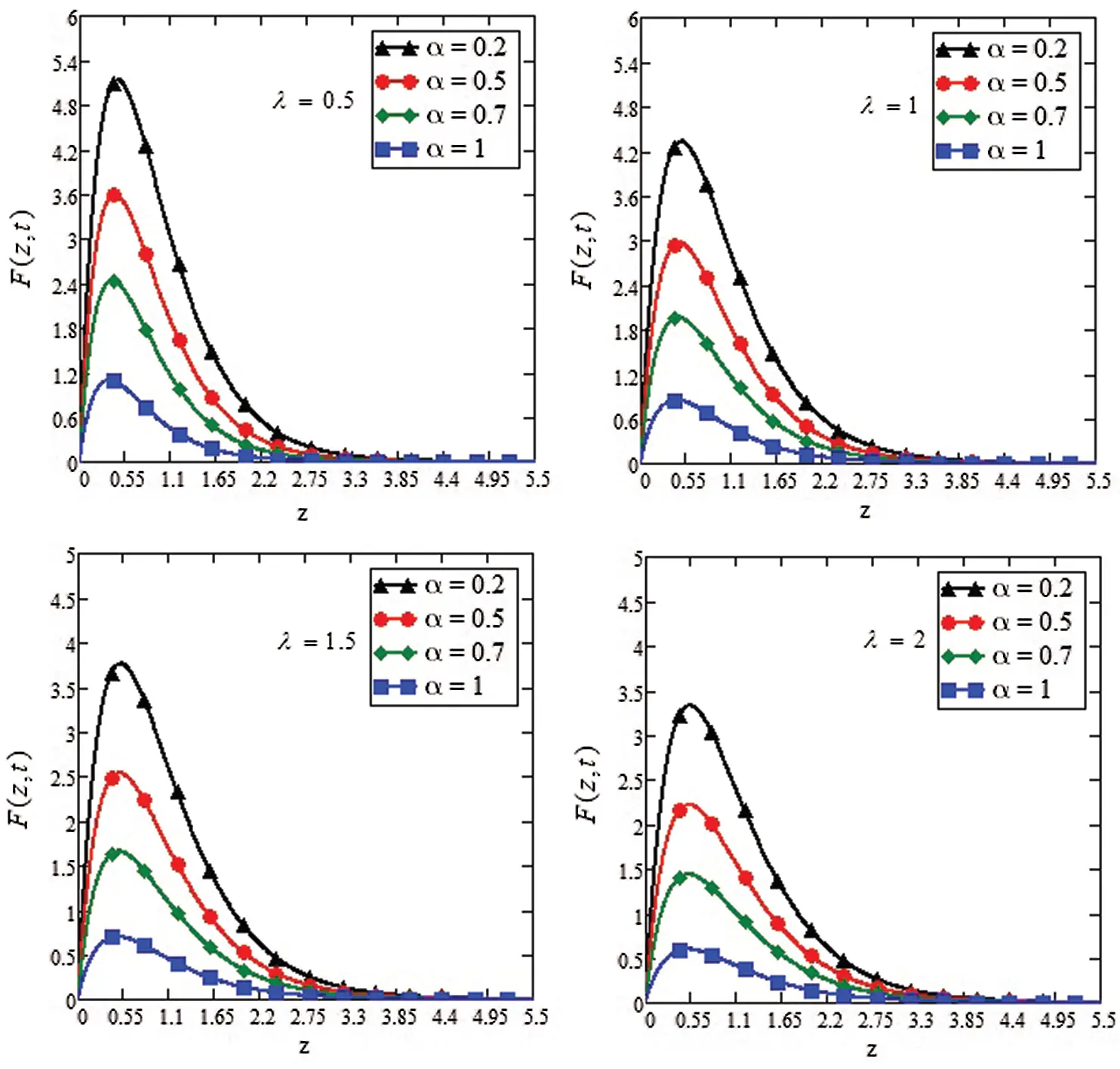Figure 5: Effect of volume fractionon the velocity profile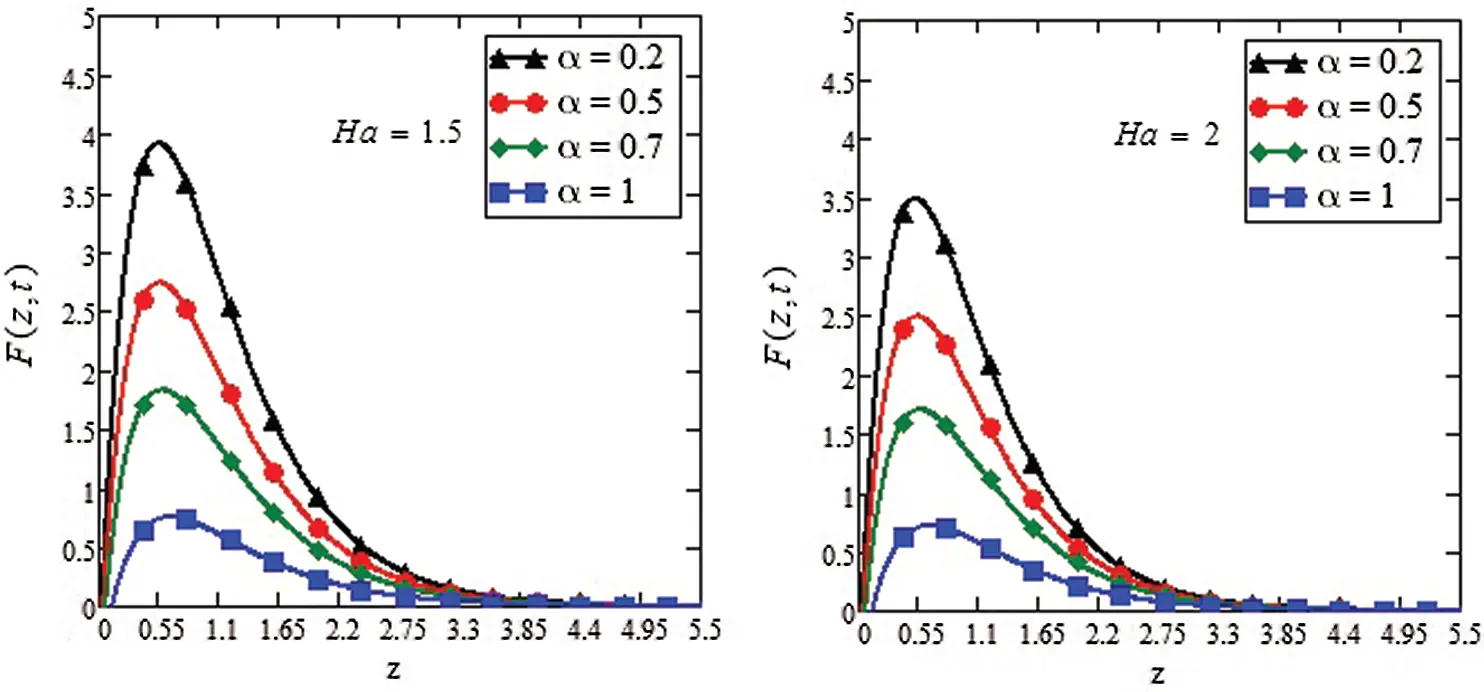Figure 6: Effect of magnetic parameter Ha on the velocity profile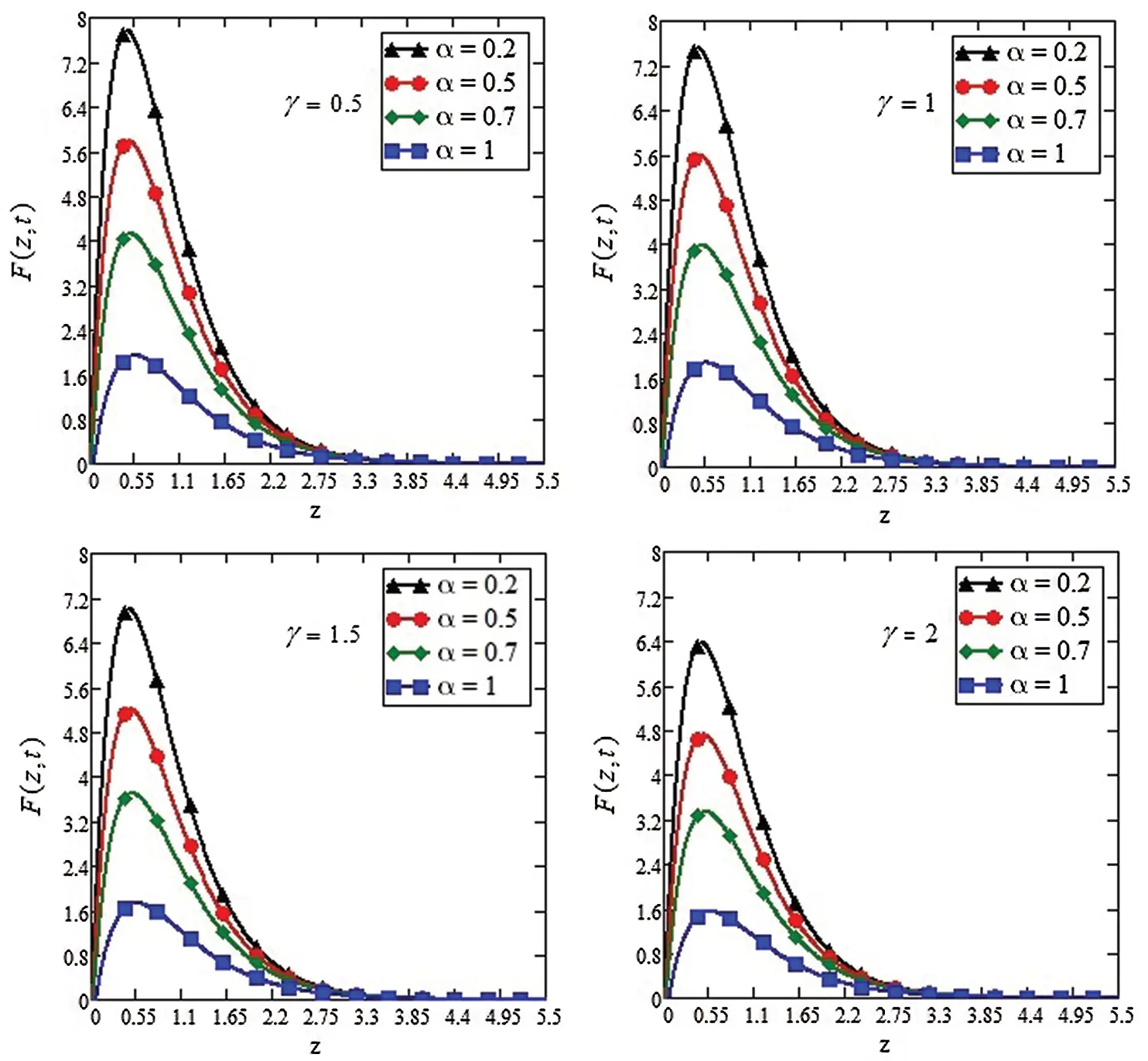Figure 7: Effect of rotation parameter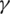on the velocity profile

Variation in the Sherwood numbers has been discussed in Tab. 2. As the volume fractionincreases, the rate of mass distribution decrease. By introducing nano-silver in the mobile oil bring enhancement in the density; as a result, the fluid becomes denser, which leads to slow down the rate of mass distribution.

Table 2: Variations in Sherwood numberTab. 3 shows a change in the rate of heat transfer of Engine oil with Silver nanoparticles. It can be seen from the table that by increasing the volume fraction of Ag nanoparticles, the heat transfer rate has been increased by 14.59%. It is physically true that by adding silver nanoparticles in regular engine oil, its mechanical properties e.g., improving the load-carrying capacity, adsorption and deposition, and minimization of skin friction which will consequently improve the workability of the engine.

Table 3: Variation in Nusselt number5  Concluding Remarks

In the present work, the role of nano-silver on the life span and mechanical properties of engine oil has been investigated. For the mentioned analysis, Jeffrey nanofluid model has been assumed in a rotating frame. The Laplace transform technique has been used to gain the solution of the governing equations. The key and worthy features that are observed from the study analysis are presented below:

•    Enhancing the order of the fractional parameter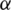slows down the flow motion.

•    Both the parameters Gr and Gm boost up the fluid motion.

•    For higher values ofandmotion of the fluid slow down.

•    The opposite behavior is noticed for MHD and Permeability.

•    As the volume fraction increase from 0.00 to 0.04, an efficiency of 14.59% in the heat transfer rate and 28.8% efficiency was reported for the mass transfer rate.

Funding Statement: The author(s) received no specific funding for this study.

Conflicts of Interest: The authors declare that they have no conflicts of interest to report regarding the present study.

## References

1. M. Waqas. (2020). “A mathematical and computational framework for heat transfer analysis of ferromagnetic non-Newtonian liquid subjected to heterogeneous and homogeneous reactions,” Journal of Magnetism and Magnetic Materials, vol. 4, no. 93, pp. 16–26.
2. A. F. Khadrawi, M. A. Al-Nimr and A. Othman. (2005). “Basic viscoelastic fluid flow problems using the Jeffrey model,” Chemical Engineering Science, vol. 60, no. 24, pp. 7131–7136.
3. T. Hayat and M. Mustafa. (2010). “Influence of thermal radiation on the unsteady mixed convection flow of a Jeffrey fluid over a stretching sheet,” Zeitschrift Für Naturforschung A, vol. 65, no. 8–9, pp. 711–719.
4. R. Ellahi, M. M. Bhatti, A. Riaz and M. Sheikholeslami. (2014). “Effects of magnetohydrodynamics on peristaltic flow of Jeffrey fluid in a rectangular duct through a porous medium,” Journal of Porous Media, vol. 17, no. 2, pp. 01–13.
5. R. Ellahi, M. M. Bhatti and I. Pop. (2016). “Effects of hall and ion slip on MHD peristaltic flow of Jeffrey fluid in a non-uniform rectangular duct,” International Journal of Numerical Methods for Heat & Fluid Flow, vol. 26, no. 6, pp. 1802–1820.
6. J. C. Maxwell, “Electricity and Magnetism,” Oxford, UK.1873: Clarendon Press.
7. S. U. Choi and J. A. Eastman. (1995). “Enhancing thermal conductivity of fluids with nanoparticles,” Argonne National Lab, vol. 1, no. 1, pp. 1–8.
8. J. A. Eastman, U. S. Choi, S. Li, L. J. Thompson and S. Lee. (1996). “Enhanced thermal conductivity through the development of nanofluids,” MRS Online Proceedings Library Archive, vol. 4, no. 57, pp. 19–26.
9. S. Dinarvand, R. Hosseini and I. Pop. (2017). “Axisymmetric mixed convective stagnation-point flow of a nanofluid over a vertical permeable cylinder by Tiwari-Das nanofluid model,” Powder Technology, vol. 3, no. 11, pp. 147–156.
10. S. T. Mohyud-Din, Z. A. Zaidi, U. Khan and N. Ahmed. (2015). “On heat and mass transfer analysis for the flow of a nanofluid between rotating parallel plates,” Aerospace Science and Technology, vol. 4, no. 6, pp. 514–522.
11. F. Ali, S. Murtaza, N. A. Sheikh and I. Khan. (2019). “Heat transfer analysis of generalized Jeffery nanofluid in a rotating frame: Atangana-Balaenu and Caputo-Fabrizio fractional models,” Chaos Solitons & Fractals, vol. 12, no. 9, pp. 1–15.
12. K. Parekh and H. S. Lee. (2010). “Magnetic field induced enhancement in thermal conductivity of magnetite nanofluid,” Journal of Applied Physics, vol. 10, no. 79, pp. 9–23.
13. P. Loganathan, C. P. Nirmal and P. Ganesan. (2013). “Radiation effects on an unsteady natural convective flow of a nanofluid past an infinite vertical plate,” Nano, vol. 8, no. 01, pp. 1–10.
14. M. Saqib, I. Khan and S. Shafie. (2018). “Application of Atangana-Baleanu fractional derivative to MHD channel flow of CMC-based-CNT’s nanofluid through a porous medium,” Chaos Solitons & Fractals, vol. 11, no. 6, pp. 79–85.
15. I. Khan, K. A. Abro, M. N. Mirbhar and I. Tlili. (2018). “Thermal analysis in Stokes’ second problem of nanofluid: Applications in thermal engineering,” Case Studies in Thermal Engineering, vol. 1, no. 2, pp. 271–275.
16. H. Alfvén. (1942). “Existence of electromagnetic-hydrodynamic waves,” Nature, vol. 150, no. 3805, pp. 405–406.
17. T. Hayat, S. Iram, T. Javed and S. Asghar. (2010). “Shrinking flow of second grade fluid in a rotating frame: An analytic solution,” Communications in Nonlinear Science and Numerical Simulation, vol. 15, no. 10, pp. 2932–2941.
18. Z. Shafique, M. Mustafa and A. Mushtaq. (2016). “Boundary layer flow of Maxwell fluid in rotating frame with binary chemical reaction and activation energy,” Results in Physics, vol. 6, no. 1, pp. 627–633.
19. G. S. Seth, R. Nandkeolyar, N. Mahto and S. K. Singh. (2009). “MHD Couette flow in a rotating system in the presence of an inclined magnetic field,” Applied Mathematical Sciences, vol. 3, no. 57–60, pp. 2919–2932.
20. S. Abelman, E. Momoniat and T. Hayat. (2009). “Steady MHD flow of a third grade fluid in a rotating frame and porous space,” Nonlinear Analysis: Real World Applications, vol. 10, no. 6, pp. 3322–3328.
21. F. Ali, M. Gohar and I. Khan. (2016). “MHD flow of water-based Brinkman type nanofluid over a vertical plate embedded in a porous medium with variable surface velocity, temperature and concentration,” Journal of Molecular Liquids, vol. 2, no. 23, pp. 412–419.
22. A. Khan, I. Khan, F. Ali and S. Shafie. (2014). “Effects of wall shear stress on unsteady MHD conjugate flow in a porous medium with ramped wall temperature,” PLoS One, vol. 9, no. 3, pp. 90–108.
23. M. D. Ortigueira and J. T. Machado. (2015). “What is a fractional derivative?” Journal of Computational Physics, vol. 29, no. 3, pp. 4–13.
24. R. Khalil, M. Al Horani, A. Yousef and M. Sababheh. (2014). “A new definition of fractional derivative,” Journal of Computational and Applied Mathematics, vol. 26, no. 4, pp. 65–70.
25. G. Jumarie. (2006). “Modified Riemann-Liouville derivative and fractional Taylor series of non-differentiable functions further results,” Computers & Mathematics with Applications, vol. 51, no. 9–10, pp. 1367–1376.
26. W. S. Chung. (2015). “Fractional Newton mechanics with conformable fractional derivative,” Journal of Computational and Applied Mathematics, vol. 2, no. 90, pp. 150–158.
27. M. Caputo and M. Fabrizio. (2015). “A new definition of fractional derivative without singular kernel,” Progression Fractional Differential Application, vol. 1, no. 2, pp. 1–13.
28. F. Ali, M. Saqib, I. Khan and N. A. Sheikh. (2016). “Application of Caputo-Fabrizio derivatives to MHD free convection flow of generalized Walters’-B fluid model,” The European Physical Journal Plus, vol. 131, no. 10, pp. 37–46.
29. F. Ali, F. Ali, N. A. Sheikh, I. Khan and K. S. Nisar. (2019). “Caputo-Fabrizio fractional derivatives modeling of transient MHD Brinkman nanoliquid: Applications in food technology,” Chaos Solitons & Fractals, vol. 10, no. 9, pp. 48–59.
30. A. Atangana and B. S. T. Alkahtani. (2016). “New model of groundwater flowing within a confine aquifer: Application of Caputo-Fabrizio derivative,” Arabian Journal of Geosciences, vol. 9, no. 1, pp. 8–17.
31. E. F. D. Goufo. (2016). “Application of the Caputo-Fabrizio fractional derivative without singular kernel to Korteweg-de Vries-Burgers equation,” Mathematical Modelling and Analysis, vol. 21, no. 2, pp. 188–198.
32. A. Atangana and D. Baleanu. (2016). “New fractional derivatives with nonlocal and non-singular kernel: Theory and application to heat transfer model,” ArXiv Preprint ArXiv, vol. 6, no. 2, pp. 40–48.
33. F. Ali, S. Murtaza, I. Khan, N. A. Sheikh and K. S. Nisar. (2019). “Atangana-Baleanu fractional model for the flow of Jeffrey nanofluid with diffusion-thermo effects, applications in engine oil,” Advances in Difference Equations, vol. 2019, no. 1, pp. 34–46.
34. S. A. A. Jan, F. Ali, N. A. Sheikh, I. Khan, M. Saqib et al. (2018). , “Engine oil based generalized brinkman-type nano-liquid with molybdenum disulphide nanoparticles of spherical shape: Atangana-Baleanu fractional model,” Numerical Methods for Partial Differential Equations, vol. 34, no. 5, pp. 1472–1488.
35. M. Saqib, F. Ali, I. Khan, N. A. Sheikh and S. B. Shafie. (2019). “Convection in ethylene glycol-based molybdenum disulfide nanofluid,” Journal of Thermal Analysis and Calorimetry, vol. 135, no. 1, pp. 523–532.
36. D. D. Gray and A. Giorgini. (1976). “The validity of the Boussinesq approximation for liquids and gases,” International Journal of Heat and Mass Transfer, vol. 19, no. 5, pp. 545–551.This work is licensed under a Creative Commons Attribution 4.0 International License, which permits unrestricted use, distribution, and reproduction in any medium, provided the original work is properly cited.